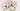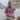# Let's Master JavaScript Function Parameters

Posted September 17, 2019Start discussion

A function is a cohesive piece of code coupled to perform a specific task. The function accesses the outer world using its parameters.

To write concise and efficient JavaScript code, you have to master the function parameters.

In this post, I will explain with interesting examples of all the features that JavaScript has to efficiently work with function parameters.

## 1. Function parameters

A JavaScript function can have any number of parameters.

Let’s define functions that have 0, 1 and 2 parameters:

``````// 0 parameters
function zero() {
return 0;
}

// 1 parameter
function identity(param) {
return param;
}

// 2 parameters
function sum(param1, param2) {
return param1 + param2;
}

zero();      // => 0
identity(1); // => 1
sum(1, 2);   // => 3``````

The 3 functions above were called with the same number of arguments as the number of parameters.

But you can call a function with fewer arguments than the number of parameters. JavaScript does not generate any errors in such a case.

However, the parameters that have no argument on invocation are initialized with `undefined` value.

For example, let’s call the function `sum()` (which has 2 parameters) with just one argument:

``````function sum(param1, param2) {
console.log(param1); // 1  console.log(param2); // undefined
return param1 + param2;
}

sum(1); // => NaN``````

The function is called only with one argument: `sum(1)`. While `param1` has the value `1`, the second parameter `param2` is initialized with `undefined`.

`param1 + param2` is evaluated as `1 + undefined`, which results in `NaN`.

If necessary, you can always verify if the parameter is `undefined` and provide a default value. Let’s make the `param2` default to `0`:

``````function sum(param1, param2) {
if (param2 === undefined) {    param2 = 0;
}
return param1 + param2;
}

sum(1); // => 1``````

But there is a better way to set default values. Let’s see how it works in the next section.

## 2. Default parameters

The ES2015 default parameters feature allows initializing parameters with defaults. It’s a better and more concise way than the one presented above.

Let’s make `param2` default to value `0` using ES2015 default parameters feature:

``````function sum(param1, param2 = 0) {  console.log(param2); // => 0
return param1 + param2;
}

sum(1);            // => 1
sum(1, undefined); // => 1``````

In the function signature there’s now `param2 = 0`, which makes `param2` default to `0` if it doesn’t get any value.

Now if the function is called with just one argument: `sum(1)`, the second parameter `param2` is initialized with `0`.

Note that if you set `undefined` as the second argument `sum(1, undefined)`, the `param2` is initialized with `0` too.

## 3. Parameter destructuring

What I especially like in the JavaScript function parameters is the ability to destructure. You can destructure inline the parameter’s objects or arrays.

This feature makes it useful to extract just a few properties from the parameter object:

``````function greet({ name }) {
return `Hello, \${name}!`;
}

const person = { name: 'John Smith' };
greet(person); // => 'Hello, John Smith!'``````

`{ name }` is a parameter on which was applied to the destructuring of an object.

You can easily combine the default parameters with destructuring:

``````function greetWithDefault({ name = 'Unknown' } = {}) {
return `Hello, \${name}!`;
}

greetWithDefault(); // => 'Hello, Unknown!'``````

`{ name = 'Unknown' } = {}` defaults to an empty object.

You can use all the power of combining the different types of destructuring. For example, let’s use the object and array destructuring on the same parameter:

``````function greeFirstPerson([{ name }]) {
return `Hello, \${name}!`;
}

const persons = [{ name: 'John Smith' }, { name: 'Jane Doe'}];
greeFirstPerson(persons); // => 'Hello, John Smith!'``````

The destructuring of parameter `[{ name }]` is more complex. It extracts the first item of the array, then reads from this item the `name` property.

## 4. arguments object

Another nice feature of JavaScript functions is the ability to call the same function with a variable number of arguments.

If you do so, it makes sense to use a special object `arguments`, which holds all the inocation arguments in an array-like object.

For example, let’s sum the arguments of a function:

``````function sumArgs() {
console.log(arguments); // { 0: 5, 1: 6, length: 2 }
let sum = 0;
for (let i = 0; i < arguments.length; i++) {
sum += arguments[i];
}
return sum;
}

sumArgs(5, 6); // => 11``````

`arguments` contains the arguments the function was called with.

`arguments` is an array-like object, so you cannot use all the fancy array methods on it.

Another difficulty is that each function scope defines it’s own `arguments` object. Thus you might need an additional variable to access the outer function scope `arguments`:

``````function outerFunction() {
const outerArguments = arguments;
return function innerFunction() {
// outFunction arguments
outerArguments;
};
}``````

### 4.1 Arrow functions case

There’s a special case: `arguments` is not available inside the arrow functions.

``````const sumArgs = () => {
console.log(arguments);
return 0;
};

// throws: "Uncaught ReferenceError: arguments is not defined"
sumArgs();``````

`arguments` is not defined inside the arrow function.

But this is not a big problem. You can use more efficiently the rest parameters to access all the arguments inside the arrow function. Let’s see how to do that in the next section.

## 5. Rest parameters

The ES2015 rest parameter lets you collect all the arguments of the function call into an array.

Let’s use the rest parameters to sum the arguments:

``````function sumArgs(...numbers) {
console.log(numbers); // [5, 6]
return numbers.reduce((sum, number) => sum + number);
}

sumArgs(5, 6); // => 11``````

`...numbers` is a rest parameter that holds the arguments in an array `[5, 6]`. Since `numbers` is an array, you can easily use all the fancy array methods on it (contrary to `arguments` that is an array-like object).

While the `arguments` object is not available inside the arrow functions, the rest parameters work without problem here:

``````const sumArgs = (...numbers) => {
console.log(numbers); // [5, 6]
return numbers.reduce((sum, number) => sum + number);
}

sumArgs(5, 6); // => 11``````

If not all the arguments should be collected inside the rest parameter, you can freely combine regular parameters with the rest parameter.

``````function multiplyAndSumArgs(multiplier, ...numbers) {
console.log(multiplier); // 2
console.log(numbers);    // [5, 6]
const sumArgs = numbers.reduce((sum, number) => sum + number);
return multiplier * sumArgs;
}

multiplyAndSumArgs(2, 5, 6); // => 22``````

`multiplier` is a regular parameter that gets the first argument’s value. Then the rest parameter `...numbers` receives the rest of the arguments.

Note that you can have up to one rest parameter per function. As well as the rest parameter must be positioned last in the function parameters list.

## 6. Conclusion

Beyond the basic usage, JavaScript offers lots of useful features when working with function parameters.

You can easily default a parameter when it’s missing.

All the power of JavaScript destructuring can be applied to parameters. You can even combine destructuring with default parameters.

`arguments` is a special array-like object that holds all the arguments the function was invoked with.

As a better alternative to `arguments`, you can use the rest parameters feature. It holds as well the arguments list, however, it stores them into an array.

Plus you can use regular parameters with rest parameter, the latter, however, must always be last in the params list.

What parameters feature do you use the most? Feel free to write a comment below!

## Quality posts into your inbox

I regularly publish posts containing:

• Important JavaScript concepts explained in simple words
• Overview of new JavaScript features
• How to use TypeScript and typing
• Software design and good coding practices